1073 and Level 1

You might not recognize it, but this puzzle is just a multiplication table. The factors in the table are all missing, and they aren’t in their usual places, but you have everything you need here to find the factors from 1 to 10 and then complete the entire multiplication table. It’s a level 1 puzzle so I am absolutely sure you can solve it!Print the puzzles or type the solution in this excel file: 10-factors-1073-1079

Here is a little information about the number 1073:

• 1073 is a composite number.
• Prime factorization: 1073 = 29 × 37
• The exponents in the prime factorization are 1 and 1. Adding one to each and multiplying we get (1 + 1)(1 + 1) = 2 × 2 = 4. Therefore 1073 has exactly 4 factors.
• Factors of 1073: 1, 29, 37, 1073
• Factor pairs: 1073 = 1 × 1073 or 29 × 37
• 1073 has no square factors that allow its square root to be simplified. √1073 ≈ 32.75668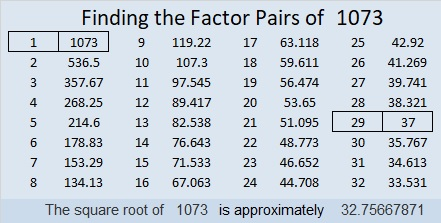32² + 7² = 1073
28² + 17² = 1073

Because 29 × 37 = 1073, it is the hypotenuse of FOUR Pythagorean triples, two of which are primitives:
348-1015-1073 which is 29 times (12-35-37)
740-777-1073 which is (20-21-29) times 37
448-975-1073 calculated from 2(32)(7), 32² – 7², 32² + 7²
495-952-1073 calculated from 28² – 17², 2(28)(17), 28² + 17²

1073 looks interesting when it is written in some other bases:
It’s 4545 in BASE 6 because 4(6³) + 5(6²) + 4(6) + 5(1) = 1073,
292 in BASE 21 because 2(21²) + 9(21) + 2(1) = 1073, and
TT in BASE 36 (T is 29 base 10) because 29(36) + 29(1) = 29(37) = 1073

997 and Level 3

Can you fill in all the cells of this 12 × 12 mixed up multiplication table if all you are given are the clues given in this puzzle? I promise you it can be done. Start with the two clues at the top of the puzzle and work down clue by clue until you have found all the factors. Afterwards, filling in the rest of the multiplication table will be a breeze.Print the puzzles or type the solution in this excel file: 12 factors 993-1001

Here are a few facts about 997, the largest three-digit prime number:

31² + 6²  = 997
That means 997 is the hypotenuse of a primitive Pythagorean triple:
372-925-997 calculated from 2(31)(6), 31² – 6², 31² + 6²

• 997 is a prime number.
• Prime factorization: 997 is prime.
• The exponent of prime number 997 is 1. Adding 1 to that exponent we get (1 + 1) = 2. Therefore 997 has exactly 2 factors.
• Factors of 997: 1, 997
• Factor pairs: 997 = 1 × 997
• 997 has no square factors that allow its square root to be simplified. √997 ≈ 31.5753

How do we know that 997 is a prime number? If 997 were not a prime number, then it would be divisible by at least one prime number less than or equal to √997 ≈ 31.3. Since 997 cannot be divided evenly by 2, 3, 5, 7, 11, 13, 17, 19, 23, 29 or 31, we know that 997 is a prime number.Here’s another way we know that 997 is a prime number: Since its last two digits divided by 4 leave a remainder of 1, and 31² + 6² = 997 with 31 and 6 having no common prime factors, 997 will be prime unless it is divisible by a prime number Pythagorean triple hypotenuse less than or equal to √997 ≈ 31.6. Since 997 is not divisible by 5, 13, 17, or 29, we know that 997 is a prime number.

877 and Level 2

29² + 6² = 877

That makes 877 the hypotenuse of a Primitive Pythagorean triple:

• 348-805-877 calculated from 2(29)(6), 29² – 6², 29² + 6²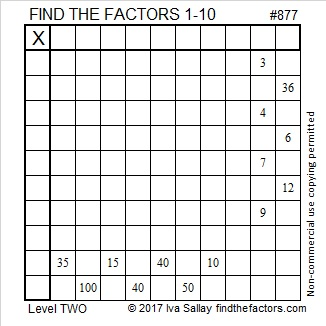Print the puzzles or type the solution on this excel file: 10-factors-875-885

• 877 is a prime number.
• Prime factorization: 877 is prime.
• The exponent of prime number 877 is 1. Adding 1 to that exponent we get (1 + 1) = 2. Therefore 877 has exactly 2 factors.
• Factors of 877: 1, 877
• Factor pairs: 877 = 1 × 877
• 877 has no square factors that allow its square root to be simplified. √877 ≈ 29.6141858

How do we know that 877 is a prime number? If 877 were not a prime number, then it would be divisible by at least one prime number less than or equal to √877 ≈ 29.6. Since 877 cannot be divided evenly by 2, 3, 5, 7, 11, 13, 17, 19, 23, or 29, we know that 877 is a prime number.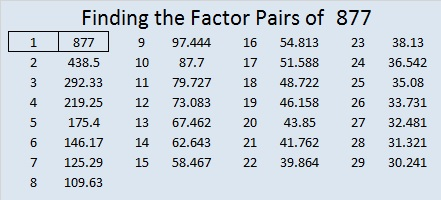Here’s another way we know that 877 is a prime number: Since its last two digits divided by 4 leave a remainder of 1, and 29² + 6² = 877 with 29 and 6 having no common prime factors, 877 will be prime unless it is divisible by a prime number Pythagorean triple hypotenuse less than or equal to √877 ≈ 29.6. Since 877 is not divisible by 5, 13, 17, or 29, we know that 877 is a prime number.

761 and Level 1

• 761 is a prime number.
• Prime factorization: 761 is prime.
• The exponent of prime number 761 is 1. Adding 1 to that exponent we get (1 + 1) = 2. Therefore 761 has exactly 2 factors.
• Factors of 761: 1, 761
• Factor pairs: 761 = 1 x 761
• 761 has no square factors that allow its square root to be simplified. √761 ≈ 27.586228.How do we know that 761 is a prime number? If 761 were not a prime number, then it would be divisible by at least one prime number less than or equal to √761 ≈ 27.6. Since 761 cannot be divided evenly by 2, 3, 5, 7, 11, 13, 17, 19, or 23, we know that 761 is a prime number.

There will be a lot about squares in today’s post, and all of the clues in today’s puzzle are perfect squares, too:Print the puzzles or type the solution on this excel file: 10 Factors 2016-02-04

—————————————

Here’s more about the number 761:

Because 761 is a prime number, there is only one way to write it as the sum of consecutive numbers: 380 + 381 = 761.

However, because it is a centered square number, 761 can be written as the sum of consecutive square numbers: 20² + 19² = 761.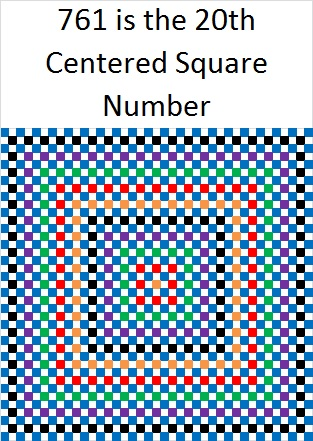And because it is the sum of two consecutive square numbers, it is also the hypotenuse of a primitive Pythagorean triple: 39-760-761 which was calculated using 20² – 19², 2(20)(19), 20² + 19².

Notice also that 20 + 19 = 39, ⌊39²/2⌋ = 760, and ⌈39²/2⌉ = 761. (Those peculiar parenthesis mean⌊round down to the closest whole number⌋ and ⌈round up to the closest whole number⌉.)

In the 39 x 39 graphic shown above there are 760 white squares and 761 squares with some color. Thus, looking at that graphic is one way to look at the primitive Pythagorean triple 39-760-761.

All of that gives us another way to know that 761 is a prime number: Since its last two digits divided by 4 leave a remainder of 1, and 20² + 19² = 761 with 20 and 19 having no common prime factors, 761 will be prime unless it is divisible by a prime number Pythagorean hypotenuse less than or equal to √761 ≈ 27.6. Since 761 is not divisible by 5, 13, or 17, we know that 761 is a prime number.

761 can also be expressed as the sum of three squares TEN ways:

• 27² + 4² + 4² = 761
• 26² + 9² + 2² = 761
• 26² + 7² + 6² = 761
• 25² + 10² + 6² = 761
• 24² + 13² + 4² = 761
• 24² + 11² + 8² = 761
• 23² + 14² + 6² = 761
• 22² + 14² + 9² = 761
• 21² + 16² + 8² = 761
• 19² + 16² + 12² = 761

761 is a palindrome in two different bases:

• 535 BASE 12; note that 5(144) + 3(12) + 5(1) = 761.
• 1I1 BASE 20 (I = 18 base 10); note that 1(400) + 18(20) + 1(1) = 761.

—————————————757 and Level 4

• 757 is a prime number.
• Prime factorization: 757 is prime.
• The exponent of prime number 757 is 1. Adding 1 to that exponent we get (1 + 1) = 2. Therefore 757 has exactly 2 factors.
• Factors of 757: 1, 757
• Factor pairs: 757 = 1 x 757
• 757 has no square factors that allow its square root to be simplified. √757 ≈ 27.51363.How do we know that 757 is a prime number? If 757 were not a prime number, then it would be divisible by at least one prime number less than or equal to √757 ≈ 27.5. Since 757 cannot be divided evenly by 2, 3, 5, 7, 11, 13, 17, 19, or 23, we know that 757 is a prime number.

Here’s today’s puzzle. A logical way to solve it can be found in a table at the end of the post.Print the puzzles or type the solution on this excel file: 12 Factors 2016-01-25

—————————————

Here’s another way we know that 757 is a prime number: Since  its last two digits divided by 4 leave a remainder of 1, and 26² + 9² = 757 with 26 and 9 having no common prime factors, 757 will be prime unless it is divisible by a prime number Pythagorean hypotenuse less than or equal to √757 ≈ 27.5. Since 757 is not divisible by 5, 13, or 17, we know that 757 is a prime number.

757 is prime so the only way it can be written as the sum of consecutive numbers is 378 + 379 = 757.

757 is the sum of seven consecutive prime numbers: 97 + 101 + 103 + 107 + 109 + 113 + 127 = 757.

From Stetson.edu we learn this distinguishing fact about the number 757: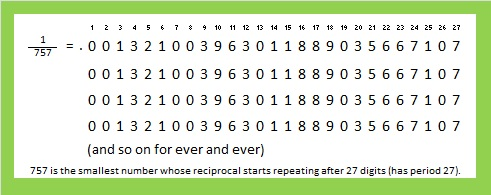Here are some square facts about the number 757:

26² + 9² = 757, and 468² + 595² = 757². That primitive Pythagorean triple, 468-595-757, can be calculated from 2(26)(9), 26² – 9², 26² + 9².

757 is also the sum of three squares two different ways:

• 24² + 10² + 9² = 757
• 18² + 17² + 12² = 757

Finally 757 is a palindrome in four different bases:

• 1001001 BASE 3; note that 1(3^6) + 0(3^5) + 0(3^4) + 1(3^3) + 0(3^2) + 0(3^1) + 1(3^0) = 757.
• 757 BASE 10; note that 7(100) + 5(10) + 7(1) = 757.
• 1F1 BASE 21 (F is 15 base 10); note that 1(21²) + 15(21) + 1(1) = 757.
• 111 BASE 27; note that 1(27²) + 1(27) + 1(1) = 757.

—————————————733 and Level 2

• 733 is a prime number.
• Prime factorization: 733 is prime.
• The exponent of prime number 733 is 1. Adding 1 to that exponent we get (1 + 1) = 2. Therefore 733 has exactly 2 factors.
• Factors of 733: 1, 733
• Factor pairs: 733 = 1 x 733
• 733 has no square factors that allow its square root to be simplified. √733 ≈ 27.0739727.

How do we know that 733 is a prime number? If 733 were not a prime number, then it would be divisible by at least one prime number less than or equal to √733 ≈ 27.1. Since 733 cannot be divided evenly by 2, 3, 5, 7, 11, 13, 17, 19, or 23, we know that 733 is a prime number.

Here’s another way we know that 733 is a prime number: Since  its last two digits divided by 4 leave a remainder of 1, and 17² + 2² = 733 with 17 and 2 having no common prime factors, 733 will be prime unless it is divisible by a prime number Pythagorean hypotenuse less than or equal to √733 ≈ 27.1. Since 733 is not divisible by 5, 13, or 17, we know that 733 is a prime number.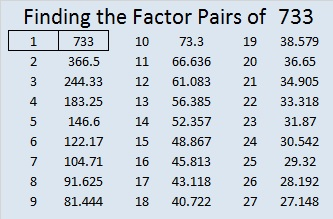Give this Level 2 factoring puzzle a try:Print the puzzles or type the solution on this excel file: 10 Factors 2016-01-04

———————————————————

Here are some more facts about the number 733:

733 is prime, so 366 + 367 = 733 is the only way it can be written as the sum of consecutive numbers.

733 is the sum of the prime numbers from 17 to 79. I think there’s a good chance you know what all those prime numbers are.

733 is also the sum of the five prime numbers from 137  to 157. You might not know what the missing primes are, but here’s a hint: If you subtract 100 from any of those three primes, you will get a composite number, and there are only three odd composite numbers that don’t end in 5 between 37 and 57.

27² + 2² = 733 so 733 is the hypotenuse of the (primitive) Pythagorean triple 108-725-733 which is calculated from 2(27)(2), 27² – 2², 27² + 2².

Thus, 108² + 725² = 733².

733 is palindrome 292 in BASE 17; note that 2(17²) + 9(17) + 2(1) = 733.

From Wikipedia I learned two other interesting facts about the number 733:

• 727, 733, 739 are consecutive prime numbers whose average is 733.  That makes 733 the 13th balanced prime. It is exactly the same distance from the previous prime and the prime that follows it.
• 337, 373, 733 are three of only nine 3-digit permutable prime numbers. No matter their order, those exact 3 digits produce a prime number.

———————————————————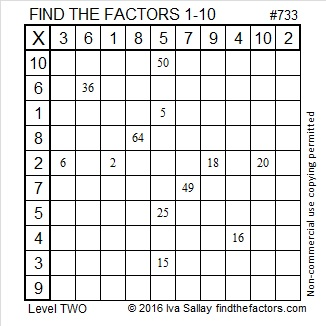701 Some Virgács left by Mikulás

• 701 is a prime number.
• Prime factorization: 701 is prime.
• The exponent of prime number 701 is 1. Adding 1 to that exponent we get (1 + 1) = 2. Therefore 701 has exactly 2 factors.
• Factors of 701: 1, 701
• Factor pairs: 701 = 1 x 701
• 701 has no square factors that allow its square root to be simplified. √701 ≈ 26.4764046.

How do we know that 701 is a prime number? If 701 were not a prime number, then it would be divisible by at least one prime number less than or equal to √701 ≈ 26.4. Since 701 cannot be divided evenly by 2, 3, 5, 7, 11, 13, 17, 19, or 23, we know that 701 is a prime number.Tonight Mikulás will visit the homes of children who sleep in Hungary. If they have been good, he will fill their boots with sweet treasures. If they have been naughty, they will receive virgács, small twigs that have been spray painted gold and bound together with red decorative ribbon. Actually most children make both the naughty list and the nice list so their boots are filled with a mixture of sweet and the not so sweet including virgács, a subtle reminder to be good.

I especially like this illustration from Wikipedia that features Mikulás (Saint Nickolas) and Krampusz:I like that it is 150 years old. It is from 1865, several decades before any of my husband’s grandparents left Hungary to live in the United States. Under the chair is a little boy hiding from Krampusz. I like to imagine he’s related to my husband some way. The little girl in the illustration must have been much better behaved that year because she is not afraid enough to need to hide.

Since everyone has been at least a little bit naughty this year, here is virgács for you to put in your shoes tonight, too.Print the puzzles or type the solution on this excel file: 12 Factors 2015-11-30

———————————————————————————

Here is a little more about the number 701:

26² + 5² = 701 so it is the hypotenuse of the primitive Pythagorean triple 260-651-701 which can be calculated using 2(26)(5), 26² – 5², 26² + 5².

701 is the sum of three consecutive prime numbers: 229 + 233+ 239.

701 is a palindrome in several bases:

• 10301 BASE 5; note that 1(625) + 0(125) + 3(25) + 0(5) + 1(1) = 701.
• 858 BASE 9; note that 8(81) + 5(9) + 8(1) = 701.
• 1F1 BASE 20; note that F is equivalent to 15 in base 10, and 1(400) + 15(20) + 1(1) = 701.
• 131 BASE 25; note that 1(625) + 3(25) + 1(1) = 701

Stetson.edu informs us that 1^0 + 2^1 + 3^2 + 4^3 + 5^4 = 701.

Here’s another way we know that 701 is a prime number: Since 701 ÷ 4 has a remainder of 1, and 701 can be written as the sum of two squares that have no common prime factors (26² + 5² = 701), then 701 will be prime unless it is divisible by a primitive Pythagorean hypotenuse less than or equal to √701 ≈ 26.4. Since 701 is not divisible by 5, 13, or 17, we know that 701 is a prime number.

———————————————————————————697 and Level 3

• 697 is a composite number.
• Prime factorization: 697 = 17 x 41
• The exponents in the prime factorization are 1 and 1. Adding one to each and multiplying we get (1 + 1)(1 + 1) = 2 x 2 = 4. Therefore 697 has exactly 4 factors.
• Factors of 697: 1, 17, 41, 697
• Factor pairs: 697 = 1 x 697 or 17 x 41
• 697 has no square factors that allow its square root to be simplified. √697 ≈ 26.40075756.Here is today’s factoring puzzle:Print the puzzles or type the solution on this excel file: 12 Factors 2015-11-30

———————————————————————————

Here are some more thoughts about the number 697:

Because both of its factors are hypotenuses of Pythagorean triples, 697 is the hypotenuse of FOUR Pythagorean triples:

• 153-680-697
• 185-672-697
• 328-615-697
• 455-528-697

Two of those triples are primitive, and two are not. Can you find the greatest common factor for each one that is not primitive?

697 is palindrome 151 in BASE 24; note that 24² = 576, and 1(576) + 5(24) + 1(1) = 697.

———————————————————————————

A Logical Approach to solve a FIND THE FACTORS puzzle: Find the column or row with two clues and find their common factor. (None of the factors are greater than 12.)  Write the corresponding factors in the factor column (1st column) and factor row (top row).  Because this is a level three puzzle, you have now written a factor at the top of the factor column. Continue to work from the top of the factor column to the bottom, finding factors and filling in the factor column and the factor row one cell at a time as you go.677 and Level 4

677 = 26² + 1², and it is the hypotenuse of the primitive Pythagorean triple 52-675-677 which was calculated using 2(26)(1),  26² – 1²,  26² + 1².

677 is also the sum of the eleven prime numbers from 41 to 83.Print the puzzles or type the solution on this excel file: 10 Factors 2015-11-09

—————————————————

• 677 is a prime number.
• Prime factorization: 677 is prime.
• The exponent of prime number 677 is 1. Adding 1 to that exponent we get (1 + 1) = 2. Therefore 677 has exactly 2 factors.
• Factors of 677: 1, 677
• Factor pairs: 677 = 1 x 677
• 677 has no square factors that allow its square root to be simplified. √677 ≈ 26.01922.How do we know that 677 is a prime number? If 677 were not a prime number, then it would be divisible by at least one prime number less than or equal to √677 ≈ 26.0. Since 677 cannot be divided evenly by 2, 3, 5, 7, 11, 13, 17, 19, or 23, we know that 677 is a prime number.

Here’s another way we know that 677 is a prime number: Since 677 ÷ 4 has a remainder of one, and 26² + 1² = 677, and 126 and 1 have no common prime factors, 677 will be prime unless it is divisible by a primitive Pythagorean hypotenuse less than or equal to √677 ≈ 26.0. Since 667 is not divisible by 5, 13, or 17, we know that 677 is a prime number.

——————————————673 and Level 6

673 = 23² + 12².

673 is the hypotenuse of the primitive Pythagorean triple 385-552-673 which was calculated using 23² – 12², 2(23)(12), 23² + 12².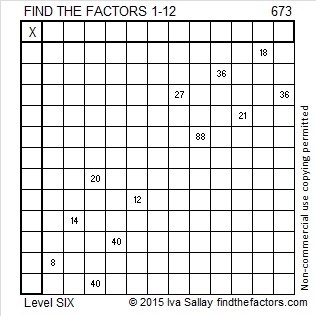Print the puzzles or type the solution on this excel file: 12 Factors 2015-11-02

—————————————————————————————————

• 673 is a prime number.
• Prime factorization: 673 is prime.
• The exponent of prime number 673 is 1. Adding 1 to that exponent we get (1 + 1) = 2. Therefore 673 has exactly 2 factors.
• Factors of 673: 1, 673
• Factor pairs: 673 = 1 x 673
• 673 has no square factors that allow its square root to be simplified. √673 ≈ 25.94224.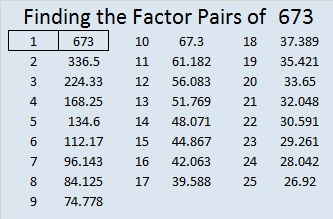How do we know that 673 is a prime number? If 673 were not a prime number, then it would be divisible by at least one prime number less than or equal to √673 ≈ 25.9. Since 673 cannot be divided evenly by 2, 3, 5, 7, 11, 13, 17, 19, or 23, we know that 673 is a prime number.

Here’s another way we know that 673 is a prime number: Since 673÷4 has a remainder of one, and 23² + 12² = 673, and 23 and 12 have no common prime factors, 673 will be prime unless it is divisible by a primitive Pythagorean hypotenuse less than or equal to √673 ≈ 25.9. Since 673 is not divisible by 5, 13, or 17, we know that 673 is a prime number.Don’t get discouraged if you make a mistake. Just fix it and move on. That’s what Ricardo did: